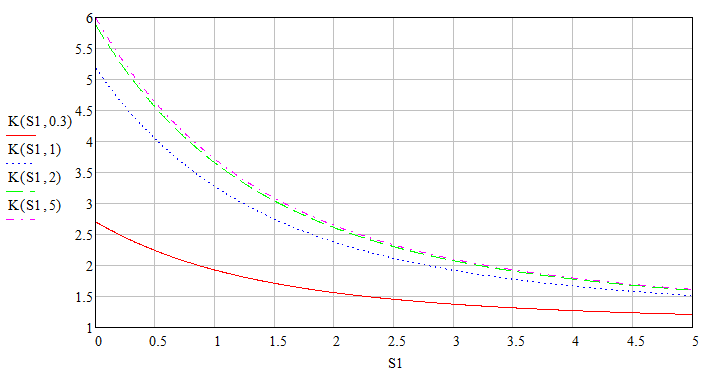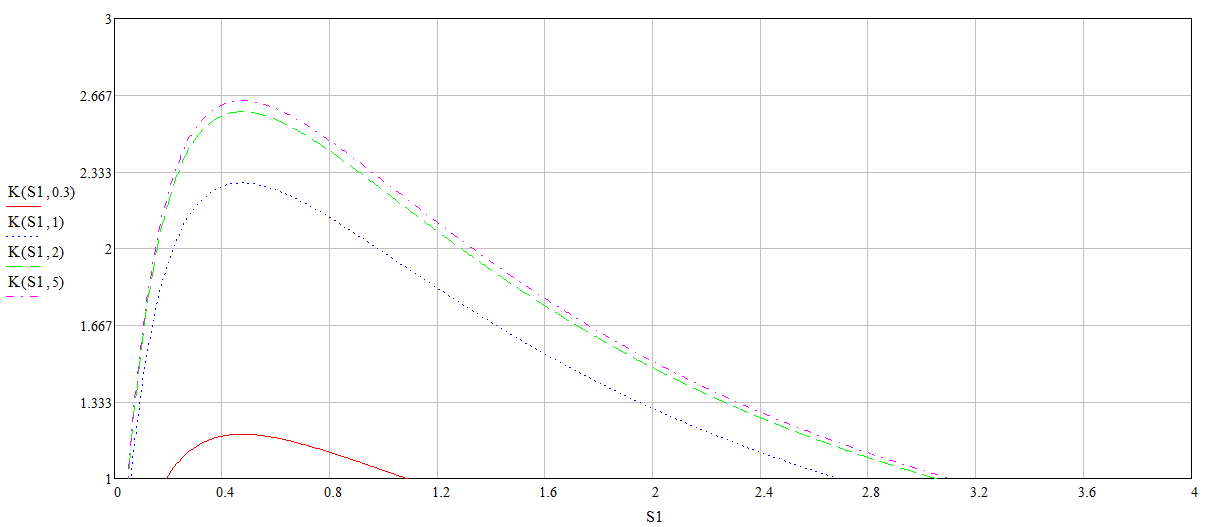Research website of Vyacheslav Gorchilin
2019-08-22
A method of increasing the efficiency of the 2nd kind on the parametric LR-circuit
Previously , we have shown the theoretical possibility of increasing the efficiency of the second kind due to the parametric change of inductance and resistance in the LR-circuit. Here, we have to consider additional factors that are always present in such schemes, bring out a more realistic formula to offer some of circuit options.
Closer to reality
The first thing we can immediately notice that the chain consisting of the $$U-R_1-L_1$$ is the circuit of the generator, which has its own internal resistance and the resistance of the inductance $$L_1$$ (Fig. 1b). Together, this will be $$R_1$$. It dissipate the losses of the generator and this energy in the formula of increase of efficiency can not be enabled. Then the formula (1.12-1.13) would transform as $K_{\eta 2} = {W_{R2} \over W_{E1}} \qquad (2.1)$ $K_{\eta 2} = \frac{\delta}{2} {\left(1 - e^{-S_1} \right)^2 \left(1 - e^{-2 S_2} \right) \over S_1 - 1 + e^{-S_1}} \qquad (2.2)$ So now we divide what we get in the load that we have spent in the source. Compare the result from the formula (1.13) presented in the first graph (Fig. 4) and the result is a more realistic formula (2.2) on the second chart (Fig. 5). The graphs shows the correlation $$K = K_{\eta 2}(S_1, S_2)$$, with $$\delta = 6$$:Fig.4. Graph of increase of efficiency of the circuit parameters for the formula (1.13)Fig.5. Graph of increase of efficiency of the circuit parameters for the formula (2.2)
The influence of key parameters and capacitance of the coil
A very big problem in these schemes are the keys SW1 and SW2, more specifically the transitional processes occurring in them, and their internal capacitance. The smaller $$S_1$$ in the latter formula, the more wag the above options to increase efficiency. Bad keys can completely neutralize this increase, as many says the famous inventor Aviso practicing this principle . Try to find out what happens in this case from the point of view of mathematics. If you look at the graph of the current in the inductor (Fig. 6a), when the transition process in the keys in this graph there will be one interval of time: $$(T_1,T_2)$$. The duration of this interval will be the time toggle key: $\tau_S = T_2 - T_1 \qquad (2.3)$Fig.6. A graph of current in inductor (a) and two concepts (b,c)
Next, we will assume that this interval is much less than the whole of the period $$(T \gg \tau_S)$$, which corresponds to the real values and simplifies calculations. Then, in the formula (2.1) dobavyatsya two terms: in the numerator — the energy loss in the switching, in the denominator of overhead in the power supply at the time of switching. $K_{\eta 2} = {K_{12}\, W_{R2} \over W_{E12} + W_{E1}} \qquad (2.4)$ $$K_{12}$$ is of the view that current in the inductor, during the $$\tau_S$$ is reduced to a value of: $I_2 = I_1 e^{-S_3}, \quad S_3 = {\tau_S \over \tau_1} \qquad (2.5)$ So: $K_{12} = e^{-2 S_3} \qquad (2.6)$ Addition in the denominator of looking at the formula (1.5), substituting there the new values: $W_{E12} = U I_1 \int \limits_0^{\tau_S} e^{-t/\tau_1} \Bbb{d}t = U I_1 \tau_1 (1 - e^{-S_3}) \qquad (2.7)$ Then the formula (2.2), taking into account transitional processes in the key will be this: $K_{\eta 2} = \frac{\delta}{2} {\left(1 - e^{-S_1} \right)^2 \left(1 - e^{-2 S_2} \right) e^{-2 S_3} \over S_1 - \left(1 - e^{-S_1} \right)e^{-S_3} } \qquad (2.8)$ The following graph shows this formula with different values for $$S_3$$, when $$\delta = 6$$. Compare it to the graph in figure (5).Fig.7. Graph of increase of efficiency of the circuit parameters. $$S_3=0.1$$Fig.8. Graph of increase of efficiency of the circuit parameters. $$S_3=0.01$$
Now let's take a closer look at the second chain $$R_2-L_2$$, which operates in a second interval (Fig. 1b). $$R_2$$ there is a load on it, so far, we considered the energy output of the entire device. But in this chain there is another resistance in the coil wire $$L_2$$, which also will dissipate a portion of energy. Let's also consider: $$R_2 = R_n + R_L$$, where $$R_n$$ is the load resistance, and $$R_L$$ resistance wire in coil $$L_2$$. Then (2.8) will be added another factor: $\Bbb{COP} = K_{\eta 2} k_L, \quad k_L = {R_n \over R_n + R_L} \qquad (2.9)$ Here and below we introduce the conversion factor $$\Bbb{COP}$$, which will show the real balance between received and expended energy. Although it only applies to heat pumps, but vplone can be used for electric generators. Its use is due to simple convenience: to distinguish between a real balance of energies and capacities from the theoretical. $$K_{\eta 2}$$ we take the formula (2.8).
The next important measure is efficiency directly to power source $$U$$ (Fig. 1b). A good source can give an efficiency close to unity: $$\eta = 0.8 .. 0.9$$, but it also needs to be taken into account when calculating: $\Bbb{COP} = K_{\eta 2} k_L \eta \qquad (2.10)$
Formula (2.10) shows us the maximum achievable result, but yet without taking into account another important parameter self-capacitance of the coil. It can greatly reduce the results and even make the release of EDS to fluctuations that will always give the result: $$K_{\eta 2} \lt 1$$. About this capacity we will discuss in the next part of this work, and now let us consider some schematic options.
Circuitry
One of the options is presented in figure (6b). The keys here work the same as in the previous case (in opposite phase), but now we can be a little more specific. Instead of SW2 can stand high-voltage high-speed diode with a low capacitance value in reverse direction (VD1). The key SW1 is likely to provide a managed high-speed semiconductor device (IGBT, thyristor), consistently with which, it may be necessary to install a protection diode (also fast). In figure (6c) shows a variant of the scheme operating in the mode of the current amplifier. Here, in the second interval the diode VD1 closes the second coil and thereby allow the excess charges in the first coil. From there, through the diode VD2, this energy goes to the load R2. In this interval the coils work like a transformer. The calculation of such a scheme is more complicated because it requires soglasovanie coils when they work in this mode. The diagrams shows a core, but need to be careful, because it can greatly distort the EMF emissions, so a more optimal coil to do without him.
Of course, in this work presents only some of the variety of circuit options; knowing the principle, you can independently develop any other, more suitable for the researcher scheme.

The materials used